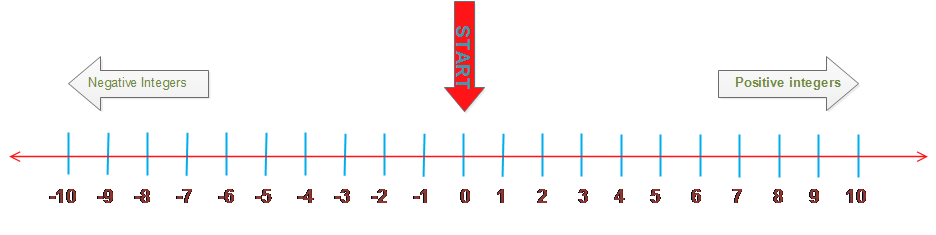# Class 7 integers word problems

Question 1
In a school exam, Rohan’s score was up to 500 points. Then he scored -100 points in the words category. What was his score then?

500-100=400 points

Question 2
Kamla has gone for a hiking trip.She ended a hike at an elevation of 5,000 feet above sea level. She had started at an elevation of 5,000 feet above sea level. Which integer represents Kamla 's change in elevation?

5000-5000=0

Question 3
Karim lives in Shrinagar , Kashmir. The table below shows the temperature changes in Shrinagar over three hour period.
 Time Change in temperature 5 a.m. to 6 a.m. -1.50C 6 a.m. to 7 a.m. -2.60C 7 a.m. to 8 a.m. +10C
Karim noticed that temperature at 5 a.m. was -3.50C . What is the temperature of Kashmir at 8 a.m.
Hint:- Start with temperature at 5 a.m. and add the change in temperature over three hour period to find the temperature at 8 a.m.

Temperature at 6= Temperature at 5 + change in temperature =-3.5 -1.5 =-5.00C
Temperature at 7= Temperature at 6 + change in temperature =-5.0 -2.6 =-7.60C
Temperature at 8= Temperature at 7 + change in temperature =-7.6 +1 =-6.60C

Question 4
Tell whether the following statements are always true, sometimes true or always false./p> a. If a positive is subtracted from a negative integer, the difference is a negative integer.
b. If a positive integer is subtracted from a positive integer, the difference is a positive integer.

a. Always true
-4 - (5) = -9 , -1 -(1) =-2
b.sometimes true
9 -4 =5 , 4 -5 =-1

Question 5 Find solutions for the following problems:
a. Both numbers are less than 10.
The distance between the two numbers on the number line is 14.
b.Both numbers are greater than -15 and less than 5
One number is 6 greater than the other number.
c.The numbers are opposites.
The distance between the two numbers on the number line is 18.
d.One number is -35.
The distance between the two numbers on the number line is 20.
e.The first number is the opposite of -17.
The second number is less than the first number.
The distance between the two numbers on the number line is 9.

These questions can be easily solved using the number line.a. There can be many solutions, one solution will be 7 and -7. They both are less 10 and difference is 14
Other solution could be -11 and 3.They both are less 10 and difference is 14
b. There can be many solutions, one solution will be -14 and -8.
Other solution could be -13 and -7.
c. This one will have unique solution. The number will be -9 and 9. Both are opposites and distance between them is 18
d. There can two solutions, one solution will be -55 and another one is -15
e. There can be one solution only. the solution is 17 and 8.